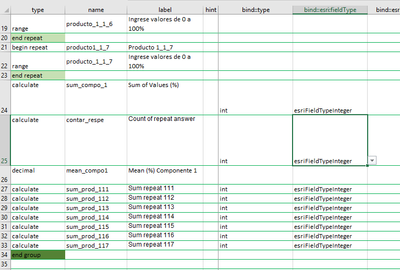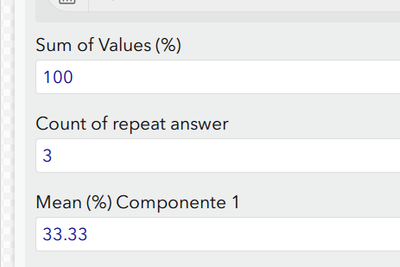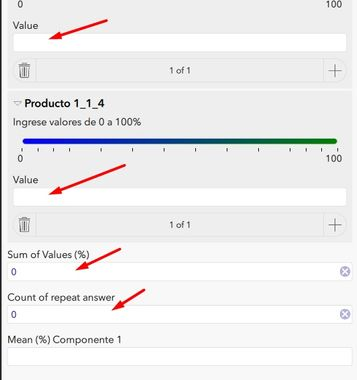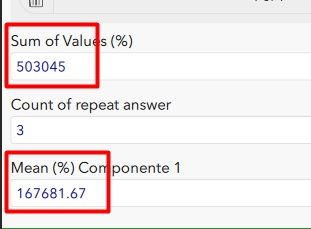# Sum and multiple repeat question, mean estimate

1491
7
12-03-2020 07:02 AMNew Contributor III

Hi,

Is have been able to sum repeated question.

However, I have an unresolved issue, I have 7 repeated integer questions, that I need to sum in order to estimate the mean.  I used the count  function so I can then estimate the mean = total sum / total count answer question.

The problem is that not always the 7 repeated questions will have a response, so that value will be NA or 0. I try to use coalesce to solve this issue, but is not working.

I don't want the 0 value counted in the count function towards estimating the mean.

I have try to get around this issue.

Example:

coalesce(sum(\${producto_1.1.1})+sum(\${producto_1.1.2})+sum(\${producto_1.1.3}),0)....

or

sum(coalesce(\${producto_1.1.1},0))+sum(coalesce(\${producto_1.1.2},0)).....

I'm attaching the XLSForm

Cheers,

Federico

2 Solutions

Accepted SolutionsbyMVP Notable Contributor

I set both bind and esri bind and it works.Bind is for within the form and esri bind is the data storage so you often need both.  Hope that helps.byEsri Regular Contributor

In addition to Doug's solution, note that you can apply the 'hidden' appearance to any question type. For example, for Sum of Values, you could use the 'integer' question type and apply the hidden appearance. The question will work like a regular integer question, just not shown on the form. When using the 'hidden' appearance, there is no need to set the bind::type or bind::esri:fieldType as these are inherited from the question type.

For the 'calculate' and 'hidden' question types the field/bind type is string by default, so Doug's solution is the way to go when using these.

7 RepliesbyMVP Notable Contributor

What exactly is not working.  Seems to work fine to me.byEsri Regular Contributor

It sounds like you want to ignore from the count any zero values (where the user has input 0 on the range slider), is that right?

The count() function counts all instances of a repeat question that contain a value (that includes 0). One option that might work is to omit the records where the value is zero using an if() statement. Please see attached example. Optionally, you could set the field type for the range questions to null to omit them from the feature layer to avoid duplicate data.

One thing to note is that with this example, if the user enters 0 for the range question, it gets converted to a blank ''. If it's important to retain the zero, an alternative could be to make the second field in the repeat a counter, using something like if(\${producto_1_1_1}>0,1,0) and then use sum() in the contar_respe field to get the total number of non-zero entries.

Best,

JimbyMVP Notable Contributor

In the sample I posted I filled out 3 of 7 fields and the count seemed to work.  That is why I asked what the exact issue is.  Range questions start as blank not 0.  Count ignores blank automatically so it all just works.New Contributor III

Dear @DougBrowning and @Jim-Moore

thanks so much for your input and help.

I uploaded the XLSForm you send and works fine; however, if I want to hide these calculations from the form e.i. Value, Sum of Values, Count of Repeat (see image below, red arrow) the calculation goes to **bleep**.

I try with "hidden" and "calculations" in the type column and also in the bind::esri:fieldType

column I selected "esriFieldTypeInteger". But no luck, I don't why this is happening?

Image 1:Image 2: when I use calculate in type column in row 8Cheers,

FedericobyMVP Notable Contributor

I set both bind and esri bind and it works.Bind is for within the form and esri bind is the data storage so you often need both.  Hope that helps.byEsri Regular Contributor

In addition to Doug's solution, note that you can apply the 'hidden' appearance to any question type. For example, for Sum of Values, you could use the 'integer' question type and apply the hidden appearance. The question will work like a regular integer question, just not shown on the form. When using the 'hidden' appearance, there is no need to set the bind::type or bind::esri:fieldType as these are inherited from the question type.

For the 'calculate' and 'hidden' question types the field/bind type is string by default, so Doug's solution is the way to go when using these.New Contributor III

Thanks @DougBrowning and @Jim-Moore

for your input and help, you guys rock!!!